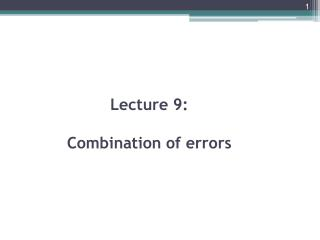# Lecture 9: Combination of errors - PowerPoint PPT PresentationDownload PresentationLecture 9: Combination of errors

Download Presentation## Lecture 9: Combination of errors

- - - - - - - - - - - - - - - - - - - - - - - - - - - E N D - - - - - - - - - - - - - - - - - - - - - - - - - - -
##### Presentation Transcript

1. Lecture 9: Combination of errors

2. Combination of errors • The determination of the value of some quantity might require several measurements to be made and their values inserted into an equation. • For example, in a determination of the density of a solid, measurements might be made of the mass m of the body and its volume V and the density calculated from m/V. The mass and volume measurements will each have errors associated with them. • How then do we determine the consequential error in the density? • The following illustrates how we can determine the worst possible error in such situations.

3. Errors when adding quantities • Consider the calculation of the quantity Z from two measured quantities A and B where Z= A +B. • If the measured quantity Ahas an error ±∆Aand the quantity B an error ± ∆ B then the worst possible error we could have in Z is if the quantities are at the extremes of their error bands and the two errors are both positive or both negative.

4. Then we have: Z+∆Z= (A+∆A) +(B+∆B), Z-∆Z = (A-∆A) +(B-∆B), • Subtracting one equation from the other gives the worst possible error as ∆Z= ∆A+∆B.

5. Errors when subtracting quantities • If we have the calculated quantity Z as the difference between two measured quantities, i.e. Z=A - B, then, in a similar way, we can show that the worst possible error is given by Z + ∆Z = (A + ∆A) - (B - ∆B), Z - ∆Z = (A - ∆A) - (B + ∆B), And so subtracting the two equations gives the worst possible error as: ∆Z = ∆A+∆B.

6. Error when multiplying quantities

7. Thus, when we have the product of measured quantities, the worst possible fractional error in the calculated quantity is the sum of the fractional errors in the measured quantities. If we multiply the above equation by 100 then: • If we have the square of a measured quantity, then all we have is the quantity multiplied by itself and so the error in the squared quantity is just twice that in the measured quantity. If the quantity is cubed then the error is three times that in the measured quantity.

8. Errors when dividing quantities • If the calculated quantity is obtained by dividing one measured quantity by another, i.e. Z = A/B, then the worst possible error is given when we have the quantities at the extremes of their error bands and the error in A positive and the error in B negative, or vice versa. Then:

9. The worst possible fractional error in the calculated quantity is the sum of the fractional errors in the measured quantities or, if expressed in percentages:

10. Example of error calculation • The above rules for determining errors when measurements are combined can be summed up: 1- When measurements are added or subtracted, the resulting worst error is the sum of the errors. 2- When measurements are multiplied or divided, the resulting worst percentage error is the sum of the percentage errors. • The following examples illustrate the use of the above to determine the worst errors.

11. Example • The distance between two points is determined from the difference between two length measurements. If these are 120 ± 0.5 mm and 230 ± 0.5 mm, what will be the error in the distance? • Answer: Adding the errors gives the difference as 110 ± 1.0 mm.

12. Example The potential difference across a resistor is measured as 2.1 ± 0.2 V and the current through it is measured as 0.25 ± 0.01 A. What will be the error in the resistance? Answer: • The percentage error in the voltage reading is (0.2/2.1) x 100% =9.5% and in the current reading is (0.01/0.25) x 100% = 4.0%. • Thusthe percentage error in the resistance is 9.5 + 4.0 = 13.5%. • Since we have V/I = 8.4 Ωand 13.5% of 8.4 is 1.1, then the resistance is 8.4 ± 1.1 Ω.

13. Example • The cross-sectional area A of awire is to be determined from a measurement of the diameter d, being given by A = . The diameter is measured as 2.5 ± 0.1 mm. What will be the error in the area?

14. Answer • The percentage error in will be twice the percentage error in d. Since the percentage error in d is ±4% then the percentage error in , and hence A since the others are pure numbers, is ±8%. • Since mm2and 8% of this value is 0.4 mm2,the result can be quoted as 4.9 ± 0.4 mm2.